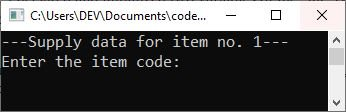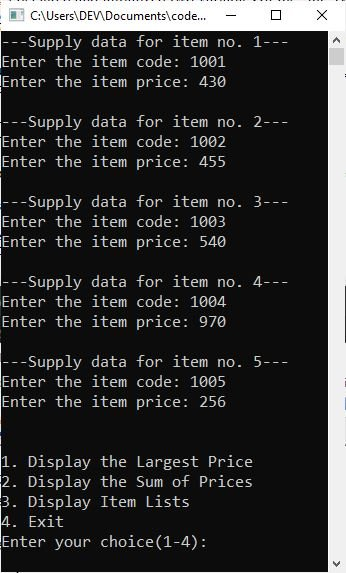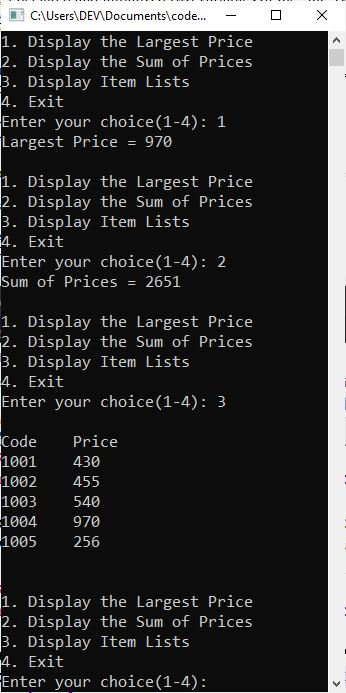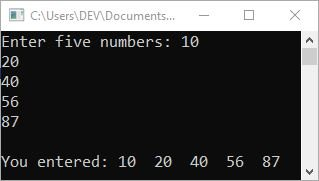# C++ Classes and Objects

This post will teach you everything you need to know about classes and objects in C++, an object-oriented feature. So, without further ado, let's get started with the "class" first.

## C++ class

As you already know, a class represents a group of similar objects. A class is a way to bind the data describing an entity and its associated functions together. For instance, consider an account having characteristics such as number, type, and balance. Deposit and withdrawal are two of its associated operations.

A class definition begins with the keyword "class," then the class name and the class body, all surrounded by curly braces. A class definition must be followed either by a semicolon or a list of declarations.

The following is the general syntax for creating a class in C++.

```class className
{
.
.
.
};```

The keyword "class" is used to create a class, and "className" is the name of the class that will be created. We define attributes and methods within and outside of the access specifiers "public," "private," and "protected" within the class. As an example:

```class CodesCracker
{
int tot;
public:
void printMsg()
{
cout<<"Sum = ";
}
void sum(int a, int b)
{
tot = a+b;
cout<<tot;
}
};```

In the above code fragment, a class named "CodesCracker" is defined, in which an "int" variable named "tot" is defined. And then two methods, namely "printMsg()" and "sum()," are defined in the "public" label.

### Class Method's Definition: Outside the scope of the class definition

The functions "printMsg()" and "sum()" in the preceding code fragment are the two member functions of the class "CodesCracker," which are defined in the class definition. However, we can also define the member function of a class later in the program instead of defining it inside the class.

A member function definition that is not part of a class definition is similar to a function definition that you are already familiar with. The only difference here is that the function name is the full name of the function, also known as the qualified name of a function, which is written as:

`class-name :: function-name`

In this case, class-name denotes that the function specified by function-name belongs to the class specified by class-name. And the symbol ::, also known as the scope resolution operator, specifies that the function's scope is limited to the class class-name. Outside of the class definition, the following is the general form of a member function definition:

```return-type class-name :: function-name(parameter list)
{
// function body
}```

### Class Declaration in C++

A class's declaration in C++ includes the declaration of its four associated attributes:

• Data members are data type properties that describe a class's characteristics. A class can have zero or more data members of any type.
• Members functions are the operations that can be performed on objects of that class. A class can have zero or more member functions.
• Program access levels govern access to members within the program. These access levels are classified as private, protected, or public.
• class tagname that serves as the class's type specifier, allowing objects of this class type to be created.

When you create a new class, you're actually creating a template for a new data type. This doesn't specify what data will be used, but it does specify what a class object is and what operations can be applied to it.

## C++ Object

When you define a class, you are not defining or creating objects of that class; rather, you are specifying the types of information that the objects of this class type will contain.

## Create C++ objects

The object of any class is declared in the same way that the basic type variables are declared. For example, the following statement declares an object of the class "CodesCracker" named "obj."

`CodesCracker obj;`

## Accessing the data members of the class in C++

Using the "dot (.)" operator, the variable (object) "obj" can now access all of the attributes and methods of the class "CodesCracker" as follows:

`obj.printMsg();`

The preceding statement called the method "printMsg()" from the class "CodesCracker." Outside of the class, attributes and members of the "PRIVATE" label are inaccessible.

Now is the time to look at an example to gain a better understanding of C++ classes and objects.

```#include <iostream>
using namespace std;

class CodesCracker
{
int tot;
public:
void printMsg()
{
cout<<"Sum = ";
}
void sum(int a, int b)
{
tot = a+b;
cout<<tot;
}
};

int main()
{
int x = 10, y = 50;

CodesCracker ob;

ob.printMsg();
ob.sum(x, y);

cout<<endl;
return 0;
}```

This program's output should be exactly:

`Sum = 60`

Let me now walk you through the above program step by step:

• I created a class named "CodesCracker."
• I declared a variable of type "int" called "total" within the class.
• Then I made a "public" label and defined two methods within it.
• That is, I created the first method, "printMsg()," which does not take an argument and does not return a value. This method only prints "Sum = " to the output console when called.
• The second method, "sum()," takes two "int" arguments, adds them, and then prints the addition result on the output console.
• Now inside the main(), I declared and initialized two variables of the "int" type, namely "x" and "y."
• Then I made a "ob" object of the class "CodesCracker."
• Now, using the object "ob," I accessed the methods "printMsg()" and "sum()."
• On the output console, the function "printMsg()" displays the text "Sum = ".
• Then I invoked the "sum()" method, passing "x" and "y" as parameters; thus, the values "x" and "y" will be copied to "a" and "b." That is, 10 and 50 will be added and printed on the output console.

## C++ Classes and Objects Example Program

Here is a practical example program demonstrating the concept of classes and objects in C++.

```#include <iostream>
using namespace std;
class ITEM
{
int itemcode;
float itprice;

public:
void initialize(void);
float largest(void);
float sum(void);
void displayitems(void);
};
void ITEM::initialize(void)
{
for (int i = 0; i < 5; i++)
{
cout << "---Supply data for item no. " << (i + 1) << "---";
cout << "\nEnter the item code: ";
cin >> itemcode[i];
cout << "Enter the item price: ";
cin >> itprice[i];
cout << "\n";
}
}
float ITEM::largest(void)
{
float larg = itprice;
for (int i = 1; i < 5; i++)
{
if (larg < itprice[i])
{
larg = itprice[i];
}
}
return larg;
}
float ITEM::sum(void)
{
float sum = 0;
for (int i = 0; i < 5; i++)
{
sum = sum + itprice[i];
}
return sum;
}
void ITEM::displayitems(void)
{
cout << "\nCode\tPrice\n";
for (int i = 0; i < 5; i++)
{
cout << itemcode[i] << "\t";
cout << itprice[i] << "\n";
}
}

int main()
{
ITEM order;
order.initialize();
float tot, big;
int ch = 0;

do
{
cout << "\n1. Display the Largest Price\n";
cout << "2. Display the Sum of Prices\n";
cout << "3. Display Item Lists\n";
cout << "4. Exit\n";
cout << "Enter your choice(1-4): ";
cin >> ch;

switch (ch)
{
case 1:
big = order.largest();
cout << "Largest Price = " << big;
break;
case 2:
tot = order.sum();
cout << "Sum of Prices = " << tot;
break;
case 3:
order.displayitems();
break;
case 4:
exit(0);
default:
cout << "\nWrong choice.";
break;
}
cout << "\n";
} while (ch >= 1 && ch <= 4);

cout<<endl;
return 0;
}```

The following snapshot depicts the above program's initial output:Now supply the inputs for five items; for example, type "1001" as an item code and hit the ENTER key, then type "430" as its price and hit the ENTER key again. Now supply the data for item number two: say "1002" as the item code and "455" as its price. In this way, supply item codes and their prices for five items. The following snapshot shows the sample run:You can now enter "1" and press the ENTER key to display the highest price, or enter 2, 3, or 4 to display the sum of prices, item lists, or exit the program. Please take a look at the image below:You can type "4" and hit the ENTER key to exit the program.

In the preceding program, I created an object "order" of the type "ITEM" (class) and used it to call the "initialize()" method, which receives five item codes and their prices, which are stored in the "itemcode" and "itprice" variables. Then I used the "do-while" loop to show the user the menu so they could select the appropriate option to get the desired result on the output console.

That is, when the user enters "1" as an option and presses the ENTER key, the class "ITEM"'s member function named "largest()" is invoked via its object "order." And this function will find the highest price among all five items' prices. If the user selects "2" or "3," I called the other two methods "sum()" and "displayitems()" in a similar manner.

Please note: Only the class's member functions have access to private members. These are not directly accessible through objects. The scope of public members, on the other hand, is determined by the referencing object. The scope of the public member is local if the referencing object is local. The scope of the public member is global if the referencing object is global.

When you need to use the value of the global variable instead of the local one, then you can use the scope resolution (::) operator. For example:

```#include <iostream>
using namespace std;
int a = 100;
int main()
{
int a = 150;
cout << "a = " << a << "\n";
cout << "::a = " << ::a << "\n\n";

{
int a = 250;
cout << "Inner Block\n";
cout << "a = " << a << "\n";
cout << "::a = " << ::a << "\n\n";
}

return 0;
}```

The output should be:

```a = 150
::a = 100

Inner Block
a = 250
::a = 100```

### C++ classes and objects: created an object array

In C++, you can make an array of objects. As an example, consider the following program:

```#include <iostream>
using namespace std;
class myClass
{
public:
int num;
};
int main()
{
myClass ob;
int i;

cout<<"Enter five numbers: ";
for(i=0; i<5; i++)
cin>>ob[i].num;

cout<<"\nYou entered: ";
for(i=0; i<5; i++)
cout<<ob[i].num<<"  ";

cout<<endl;
return 0;
}```

The following snapshot shows the sample run of the above program with user inputs of 10, 20, 40, 56, and 87 as five number inputs.C++ Quiz

« Previous Tutorial Next Tutorial »